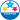Highlighted
New Member

Can someone explain how a final grade is determined if a teacher chooses category weighting?

For example, 2 categories. Classwork = 30% and Assessment = 70%

In the traditional grade settings it is set to calculate a final grade based on those categories.

HighlightedPowerSchool Champion

Hi @rcox ,

That calculation will take the average of the scores in the classwork category and multiply it by .30 and the avergae of the scores in the assessment category and multiply it by .70, then add those scores together.

I am not great at explaining math, so an example will make this clearer:

Scores in Classwork:

85/100

65/100

78/100=

228/300=76% * .3=22.8

Scores in Assessment:

55/100

23/50

67/100

85/100=

230/350=66% * .7=46.2

Score for class= 22.8+46.2=69%

______________
Full Disclosure: I do not work for PowerSchool